# A Beautiful Equation

$$e^{i\pi} + 1 = 0$$

Euler gave us this jewel which is regarded by many as the most beautiful equation in mathematics; it has both elegance and simplicity.

It is comprised of five fundamental numbers and the operations of addition, multiplication, exponentiation (raising to a power) and equality.

The numbers, in order of mathematical discovery :

$$1$$

One is unity.  It is the first of the natural (counting) numbers, $$\Bbb N$$.  It is positive and odd.  It is neither prime nor composite.

It is the multiplicative identity since $$1 \times x = x \times 1 = x$$.

$$\pi$$

“How I want a drink, alcoholic of course, after the heavy lectures …”

$$3.14159 \, 26535 \, 8 …$$

Perhaps the most famous number in mathematics which used to be known as Archimedes’ constant.  The lowercase Greek letter, pi, (made popular by Euler) is used to denote the constant that is the ratio of the circumference of a circle to its diameter.

$$\displaystyle \pi = \frac Cd$$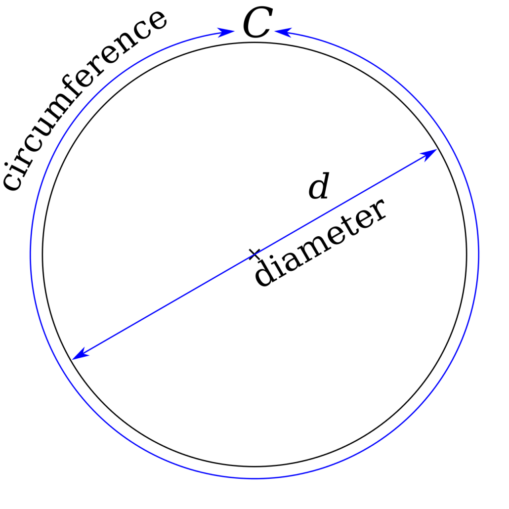The circumference of a circle is slightly more than three times as long as its diameter. The exact ratio is called πKjoonlee, based on previous work by w:User:Papeschr [Public domain], via Wikimedia Commons

Archimedes (c. 225 BC)  used the approximation $$22/7$$ which should be known by every schoolchild.

$$\pi$$ is irrational (proved by Lambert, 1768), that is, it cannot be represented as a fraction and belong to the rational numbers, $$\Bbb Q$$.  It is a real number, $$\Bbb R$$.

$$\pi$$ is transcendental (proved by Lindemann, 1882), that is, it cannot be a root of any algebraic equation with rational coefficients.  Thus, one cannot square the circle.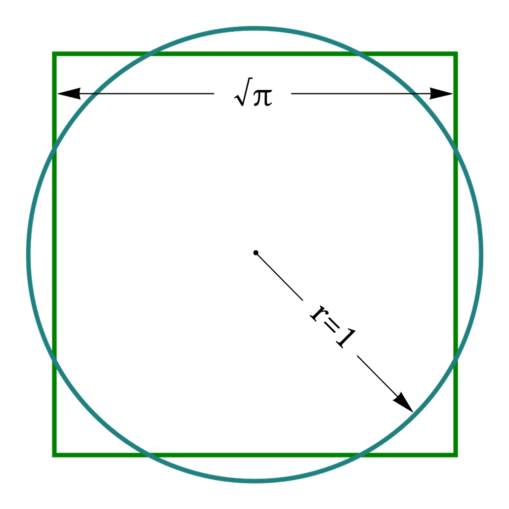Because π is a transcendental number, squaring the circle is not possible in a finite number of steps using the classical tools of compass and straightedgeOriginal PNG by Plynn9; SVG by Alexei Kouprianov [Public domain], via Wikimedia Commons

It has the infinite continued fraction $$[3; 7,15,1,292,1,1,1,2,1,3,1,4,2,1,1,…]$$.

Apart from geometry, $$\pi$$ is found in many other areas such as trigonometry, number theory and statistics.  Radians are used as the units of angular measurement and there are $$2\pi$$ radians in the revolution of a circle.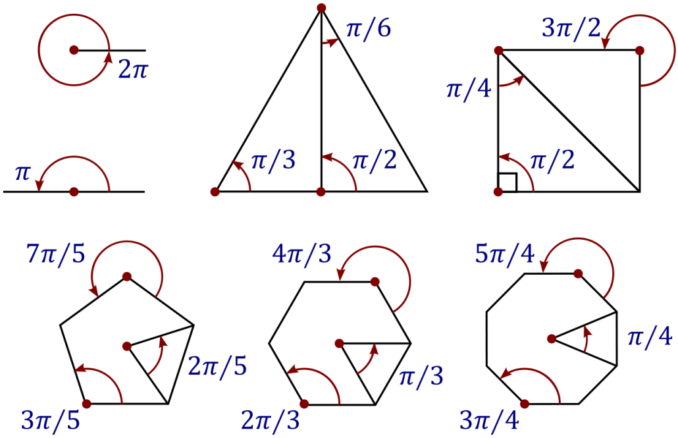Some common angles, measured in radians. All the large polygons in this diagram are regular polygonsTraced by User:Stannered [CC BY-SA 3.0], via Wikimedia Commons

$$0$$

For a long time zero was considered merely as nothing or as a placeholder.  In $$628$$ Brahmagupta defined zero as $$a-a = 0$$ and thus as a number in its own right.

Zero is even.  It is an integer number, $$\Bbb Z$$ , which is neither positive nor negative and is the smallest non-negative integer.  It is sometimes sometimes used for counting where one puts it in $$\Bbb N^+$$, the natural numbers plus zero.  One may choose to start counting at $$1$$ or $$0$$.

Zero is the additive identity since $$x + 0 = 0 + x = x$$.

Up to the real numbers, $$\Bbb R$$, division by zero is undefined.  In the complex numbers, $$\Bbb C$$, division by zero can be considered as defined in some contexts.  It is the point at complex infinity in the extended complex plane, $$\Bbb {\hat C}$$ (the Riemann sphere).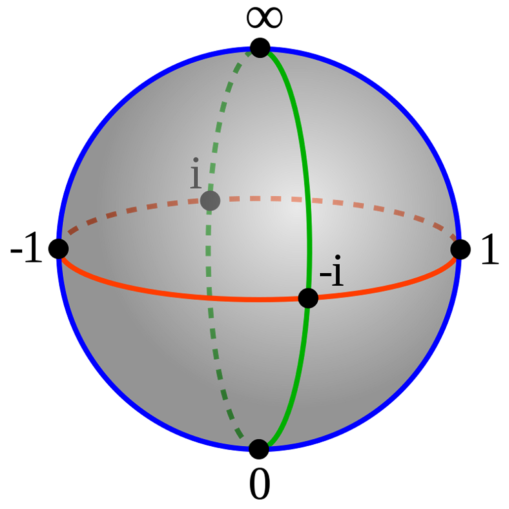The Riemann sphere can be visualized as the complex number plane wrapped around a sphereGKFXtalk, de:User:Bjoern_klipp [CC BY-SA 3.0], via Wikimedia Commons

$$i$$

$$i$$ belongs to the complex numbers, $$\Bbb C$$.  Complex numbers take the form $$z = a + bi$$ which consists of a real part, $$a$$, and an imaginary part, $$bi$$.  If $$a = 0$$, then the number is purely imaginary and lies somewhere on the Im axis.  If $$b = 0$$, then the number is purely real and lies on the Re axis.   Complex numbers in the complex plane are neither positive nor negative.

Complex numbers satisfy the normal rules of arithmetic with the further rule that states :

$$i^2 =-1$$

There are two roots to this equation since both $$i^2$$ and $$(-i)^2$$ equal $$-1$$, however, one of them needs to be defined as “the” imaginary unit.  Either choice is possible; we choose to define $$i$$ as “the” imaginary unit and hence “the” square root of $$-1$$.

$$i = \sqrt-1$$

Multiplication of a complex number by $$i$$ produces a rotation through $$\pi/2$$ (radians) about the origin ($$0$$) in a counter-clockwise direction.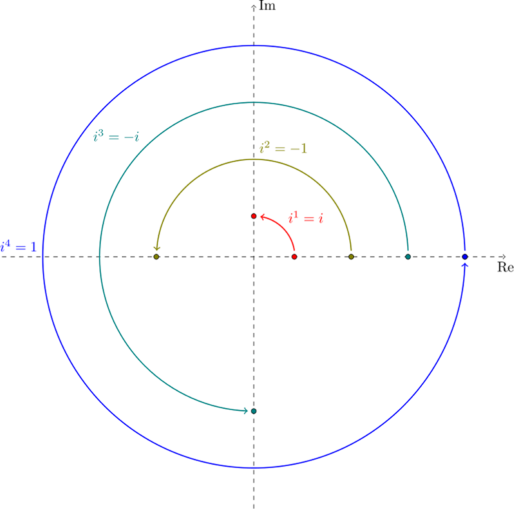90-degree rotations in the complex planeKeφr [CC0], via Wikimedia Commons

$$e$$

“To express $$e$$, remember to memorise a sentence to simplify this . . .”

$$2.71828\, 18284\, …$$

$$e$$ is the base of the natural logarithm and was known as Napier’s constant until Euler denoted it as $$e$$.  It is found wherever growth is involved.

It can be evaluated in various ways.

As a limit as $$n$$ approaches infinity :

$$\displaystyle e = \lim_{n\to \infty} \left (1 + \frac 1n \right)^n$$

As an infinite sum (where $$n!$$ is the factorial function) :

$$\displaystyle e = \sum_{n=0}^\infty \frac {1}{n!} = \frac {1}{0!} + \frac {1}{1!} + \frac {1}{2!} + \frac {1}{3!} + \frac {1}{4!} + \frac {1}{5!} + . . .$$

$$e$$ is irrational (Euler, 1737) and transcendental (Hermite, 1873).  It belongs to the real numbers, $$\Bbb R$$.

It has the infinite continued fraction $$[2; 1, 2, 1, 1, 4, 1, 1, 6, 1, 1, 8, 1, 1, 10, 1,…1, 2n, 1, …]$$.

Euler’s equation is a special case of Euler’s formula which relates the complex exponential function to the trigonometric cosine and sine functions.

For any real number $$x$$ (in radians) :

$$e^{ix} = \cos x + i\sin x$$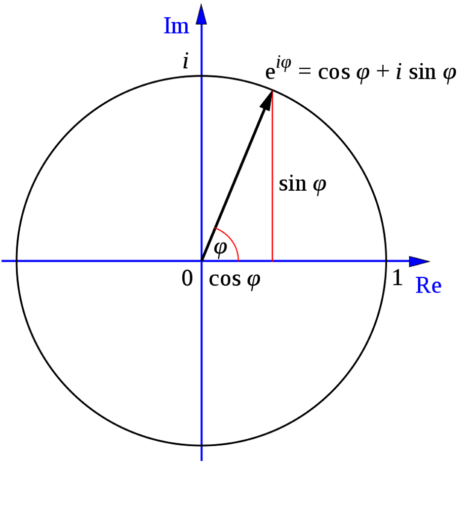Euler’s formula illustrated in the complex planeOriginal: GuntherDerivative work: Wereon [CC BY-SA 3.0], via Wikimedia Commons

When $$x = \pi$$,

$$e^{i\pi} = -1 + i \times 0$$,

since $$\cos \pi = -1$$ and $$\sin \pi = 0$$.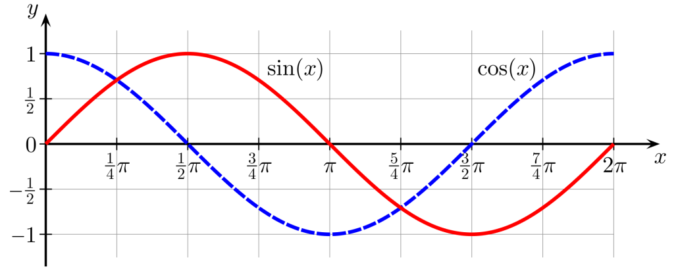Sine and cosine functions repeat with period 2πGeek3 [CC BY 3.0], via Wikimedia Commons

Rearranging gives :

$$e^{i\pi} + 1 = 0$$

© OldTrout $$2019$$